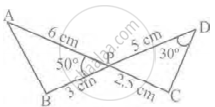# In figure, two line segments AC and BD intersect each other at the point P such that PA = 6 cm, PB = 3 cm, PC = 2.5 cm, PD = 5 cm, ∠APB = 50° and ∠CDP = 30°. Then, ∠PBA is equal to ______. - Mathematics

MCQ
Fill in the Blanks

In figure, two line segments AC and BD intersect each other at the point P such that PA = 6 cm, PB = 3 cm, PC = 2.5 cm, PD = 5 cm, ∠APB = 50° and ∠CDP = 30°. Then, ∠PBA is equal to ______.• 50°

• 30°

• 60°

• 100°

#### Solution

In figure, two line segments AC and BD intersect each other at the point P such that PA = 6 cm, PB = 3 cm, PC = 2.5 cm, PD = 5 cm, ∠APB = 50° and ∠CDP = 30°. Then, ∠PBA is equal to 100°.Explanation:

From ∆APB and ∆CPD,

∠APB = ∠CPD = 50° ......(Since they are vertically opposite angles)

(AP)/(PD) = 6/5  ......(i)

Also, (BP)/(CP) = 3/2.5

Or (BP)/(CP) = 6/5  ......(ii)

From equations (i) and (ii),

We get,

(AP)/(PD) = (BP)/(CP)

So, ∆APB ∼ ∆DPC  ......[Using SAS similarity criterion]

∴ ∠A = ∠D = 30°  ......[Since, corresponding angles of similar triangles]

Since, Sum of angles of a triangle = 180°,

In ∆APB,

∠A + ∠B + ∠APB = 180°

So, 30° + ∠B + 50° = 180°

Then, ∠B = 180° – (50° + 30°)

∠B = 180 – 80° = 100°

Therefore, ∠PBA = 100°

Concept: Similarity of Triangles
Is there an error in this question or solution?

#### APPEARS IN

NCERT Mathematics Exemplar Class 10
Chapter 6 Triangles
Exercise 6.1 | Q 5 | Page 61

Share